Advertisement Remove all ads

# SCERT Maharashtra Question Bank solutions for 10th Standard SSC Mathematics 2 Geometry Maharashtra State Board 2021 chapter 1 - Similarity [Latest edition]

Advertisement Remove all ads

#### Chapters## Chapter 1: Similarity

Q.1 (A)Q.1 (B)Q.2 (A)Q.2 (B)Q.3 (A)Q.3 (B)Q.4Q.5
Q.1 (A)

### SCERT Maharashtra Question Bank solutions for 10th Standard SSC Mathematics 2 Geometry Maharashtra State Board 2021 Chapter 1 Similarity Q.1 (A)

#### MCQ [1 Mark]

Q.1 (A) | Q 1

If ∆ABC ~ ∆PQR and AB : PQ = 3 : 4 then A(∆ABC) : A(∆PQR) = ?

• 9 : 25

• 9 : 16

• 16 : 9

• 25 : 9

Q.1 (A) | Q 2

Which of the following is not a test of similarity?

• AAA

• SAS

• SAA

• SSS

Q.1 (A) | Q 3

If ∆XYZ ~ ∆PQR and A(∆XYZ) = 25 cm2, A(∆PQR) = 4 cm2 then XY : PQ = ?

• 4 : 25

• 2 : 5

• 5 : 2

• 25 : 4

Q.1 (A) | Q 4

Ratio of areas of two similar triangles is 9 : 25. _______ is the ratio of their corresponding sides.

• 3 : 4

• 3 : 5

• 5 : 3

• 25 : 81

Q.1 (A) | Q 5

Given ΔABC ~ ΔDEF, if ∠A = 45° and ∠E = 35° then ∠B = ?

• 45°

• 35°

• 25°

• 40°

Q.1 (A) | Q 6

In fig, seg DE || sec BC, identify the correct statement.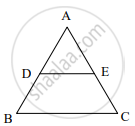• "AD"/"DB" = "AE"/"AC"

• "AD"/"DB" = "AB"/"AC"

• "AD"/"DB" = "EC"/"AC"

• "AD"/"DB" = "AE"/"EC"

Q.1 (A) | Q 7

If ΔXYZ ~ ΔPQR then "XY"/"PQ" = "YZ"/"OR" = ?

• "XZ"/"PR"

• "XZ"/"PQ"

• "XZ"/"QR"

• "YZ"/"PQ"

Q.1 (A) | Q 8

If ΔABC ~ ΔLMN and ∠A = 60° then ∠L = ?

• 45°

• 60°

• 25°

• 40°

Q.1 (A) | Q 9

In ΔDEF and ΔXYZ, "DE"/"XY" = "FE"/"YZ" and ∠E ≅ ∠Y. _______ test gives similarity between ΔDEF and ΔXYZ.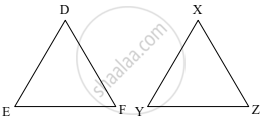• AAA

• SAS

• SAA

• SSS

Q.1 (A) | Q 10

In fig. BD = 8, BC = 12, B-D-C, then ("A"(Δ"ABC"))/("A"(ΔABD")) = ?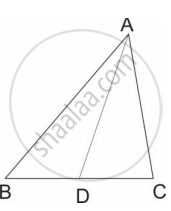• 2 : 3

• 3 : 2

• 5 : 3

• 3 : 4

Advertisement Remove all ads
Q.1 (B)

### SCERT Maharashtra Question Bank solutions for 10th Standard SSC Mathematics 2 Geometry Maharashtra State Board 2021 Chapter 1 Similarity Q.1 (B)

#### Solve [1 Mark]

Q.1 (B) | Q 1

Are triangles in figure similar? If yes, then write the test of similarity.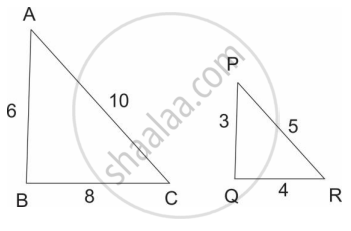Q.1 (B) | Q 2

In fig., line BC || line DE, AB = 2, BD = 3, AC = 4 and CE = x, then find the value of x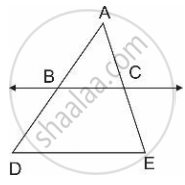Q.1 (B) | Q 3

State whether the following triangles are similar or not: If yes, then write the test of similarity.

∠P = 35°, ∠X = 35° and ∠Q = 60°, ∠Y = 60°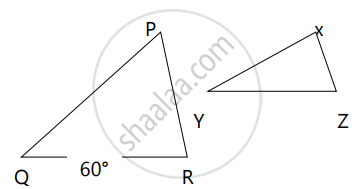Q.1 (B) | Q 4

If ΔABC ~ ΔLMN and ∠B = 40°, then ∠M = ? Give reason.

Q.1 (B) | Q 5

Areas of two similar triangles are in the ratio 144:49. Find the ratio of their corresponding sides

Q.1 (B) | Q 6

ΔPQR ~ ΔSUV. Write pairs of congruent angles

Q.1 (B) | Q 7

ΔABC ~ ΔDEF. Write the ratios of their corresponding sides

Q.1 (B) | Q 8

In fig., TP = 10 cm, PS = 6  "cm"* ("A"(Δ"RTP"))/("A"(Δ"RPS")) = ?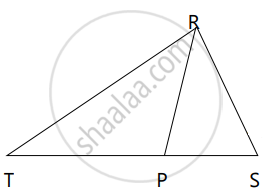Q.1 (B) | Q 9

Ratio of corresponding sides of two similar triangles is 4:7, then find the ratio of their areas = ?

Q.1 (B) | Q 10

Write the test of similarity for triangles given in figure.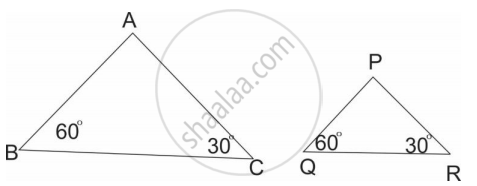Advertisement Remove all ads
Q.2 (A)

### SCERT Maharashtra Question Bank solutions for 10th Standard SSC Mathematics 2 Geometry Maharashtra State Board 2021 Chapter 1 Similarity Q.2 (A)

#### Complete the activity [2 Marks]

Q.2 (A) | Q 1

In fig. BP ⊥ AC, CQ ⊥ AB, A−P−C and A−Q−B then show that ΔAPB and ΔAQC are similar.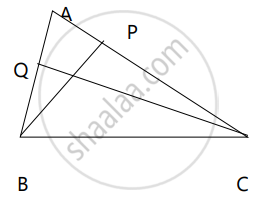In ΔAPB and ΔAQC

∠APB = [   ]°     ......(i)

∠AQC = [   ]°  ......(ii)

∠APB ≅ ∠AQC    .....[From (i) and (ii)]

∠PAB ≅ ∠QAC    .....[______]

ΔAPB ~ ΔAQC     .....[______]

Q.2 (A) | Q 2

Observe the figure and complete following activity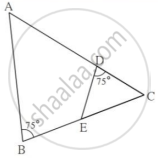In fig, ∠B = 75°, ∠D = 75°

∠B ≅ [______]       ......[each of 75°]

∠C ≅ ∠C          .......[______]

ΔABC ~ Δ [______]     ......[______ similarity test]]

Q.2 (A) | Q 3

ΔABC ~ ΔPQR, A(ΔABC) = 80 sq.cm, A(ΔPQR) = 125 sq.cm, then complete ("A"(Δ"ABC"))/("A"(Δ"PQR")) = 80/125 = (["______"])/(["______"]), hence "AB"/"PQ" = (["______"])/(["______"])

Q.2 (A) | Q 4

In fig., PM = 10 cm, A(ΔPQS) = 100 sq.cm, A(ΔQRS) = 110 sq.cm, then NR?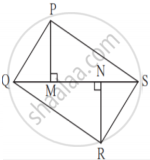ΔPQS and ΔQRS having seg QS common base.

Areas of two triangles whose base is common are in proportion of their corresponding [______]

("A"("PQS"))/("A"("QRS")) = (["______"])/"NR",

100/110 = (["______"])/"NR",

NR = [______] cm

Advertisement Remove all ads
Q.2 (B)

### SCERT Maharashtra Question Bank solutions for 10th Standard SSC Mathematics 2 Geometry Maharashtra State Board 2021 Chapter 1 Similarity Q.2 (B)

#### [2 Marks]

Q.2 (B) | Q 1

In fig., AB ⊥ BC and DC ⊥ BC, AB = 6, DC = 4 then ("A"(Δ"ABC"))/("A"(Δ"BCD")) = ?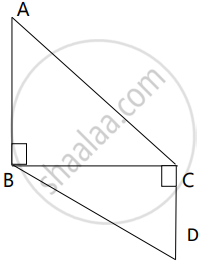Q.2 (B) | Q 2

In fig., seg AC and seg BD intersect each other at point P.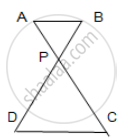"AP"/"PC" = "BP"/"PD" then prove that ΔABP ~ ΔCDP

Q.2 (B) | Q 3

ΔABP ~ ΔDEF and A(ΔABP) : A(ΔDEF) = 144:81, then AB : DE = ?

Q.2 (B) | Q 4

From given information, is PQ || BC?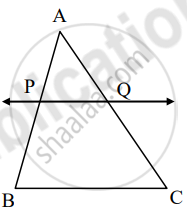AP = 2, PB = 4, AQ = 3, QC = 6

Q.2 (B) | Q 5

Areas of two similar triangles are 225 cm2 and 81 cm2. If side of smaller triangle is 12 cm, find corresponding side of major triangle

Q.2 (B) | Q 6

From adjoining figure, ∠ABC = 90°, ∠DCB = 90°, AB = 6, DC = 8, then ("A"(Δ"ABC"))/("A"(Δ"BCD")) = ?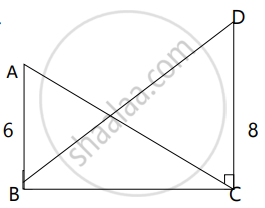Advertisement Remove all ads
Q.3 (A)

### SCERT Maharashtra Question Bank solutions for 10th Standard SSC Mathematics 2 Geometry Maharashtra State Board 2021 Chapter 1 Similarity Q.3 (A)

#### Complete the following activities [3 Marks]

Q.3 (A) | Q 1

In DABC, AP ⊥ BC and BQ ⊥ AC, B−P−C, A−Q−C, then show that ΔCPA ~ ΔCQB. If AP = 7, BQ = 8, BC = 12, then AC = ?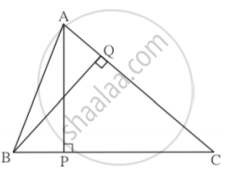In ΔCPA and ΔCQB

∠CPA ≅ [∠ ______]    .......[each 90°]

∠ACP ≅ [∠ ______]   .......[common angle]

ΔCPA ~ ΔCQB     ......[______ similarity test]

"AP"/"BQ" = (["______"])/"BC"    .......[corresponding sides of similar triangles]

7/8 = (["______"])/12

AC × [______] = 7 × 12

AC = 10.5

Q.3 (A) | Q 2

A line is parallel to one side of triangle which intersects remaining two sides in two distinct points then that line divides sides in same proportion.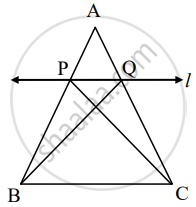Given: In ΔABC line l || side BC and line l intersect side AB in P and side AC in Q.

To prove: "AP"/"PB" = "AQ"/"QC"

Construction: Draw CP and BQ

Proof: ΔAPQ and ΔPQB have equal height.

("A"(Δ"APQ"))/("A"(Δ"PQB")) = (["______"])/"PB"   .....(i)[areas in proportion of base]

("A"(Δ"APQ"))/("A"(Δ"PQB")) = (["______"])/"QC"  .......(ii)[areas in proportion of base]

ΔAPQ and ΔPQB have [______] is common base.

Seg PQ || Seg BC, hence height of ΔAPQ and ΔPQB.

A(ΔPQC) = A(Δ______)    ......(iii)

("A"(Δ"APQ"))/("A"(Δ"PQB")) = ("A"(Δ "______"))/("A"(Δ "______"))   ......[(i), (ii), and (iii)]

"AP"/"PB" = "AQ"/"QC"   .......[(i) and (ii)]

Q.3 (A) | Q 3

From fig., seg PQ || side BC, AP = x + 3, PB = x – 3, AQ = x + 5, QC = x – 2, then complete the acitivity to find the value of x.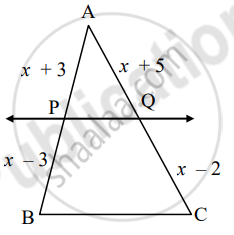In ΔPQB, PQ || side BC

"AP"/"PB" = "AQ"/(["______"])    .......[______]

(x + 3)/(x - 3) = (x + 5)/(["______"])

(x + 3) [______] = (x + 5)(x – 3)

x2 + x – [______] = x2 + 2x – 15

x = [______]

Advertisement Remove all ads
Q.3 (B)

### SCERT Maharashtra Question Bank solutions for 10th Standard SSC Mathematics 2 Geometry Maharashtra State Board 2021 Chapter 1 Similarity Q.3 (B)

#### [3 Marks]

Q.3 (B) | Q 1

There are two poles having heights 8 m and 4 m on plane ground as shown in fig. Because of sunlight shadows of smaller pole is 6m long, then find the length of shadow of longer pole.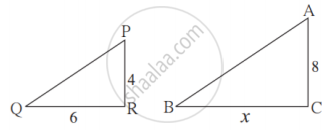Q.3 (B) | Q 2. 1)

In ΔABC, B − D − C and BD = 7, BC = 20, then find the following ratio.

("A"(Δ"ABD"))/("A"(Δ"ADC")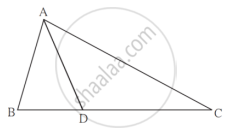Q.3 (B) | Q 2. 2)

In ΔABC, B − D − C and BD = 7, BC = 20, then find the following ratio.

("A"(Δ"ABD"))/("A"(Δ"ABC")Q.3 (B) | Q 2. 3)

In ΔABC, B − D − C and BD = 7, BC = 20, then find the following ratio.

("A"(Δ"ADC"))/("A"(Δ"ABC")Q.3 (B) | Q 3

In given fig., quadrilateral PQRS, side PQ || side SR, AR = 5 AP, then prove that, SR = 5PQ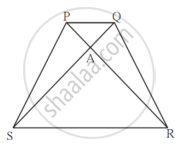Q.3 (B) | Q 4

In triangle ABC point D is on side BC(B − D − C) such that ∠BAC = ∠ADC then prove that CA2 = CB × CD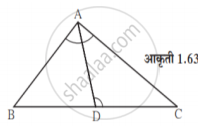Q.3 (B) | Q 5

In Quadrilateral ABCD, side AD || BC, diagonal AC and BD intersect in point P, then prove that "AP"/"PD" = "PC"/"BP"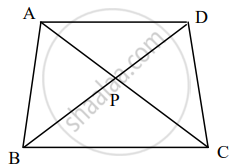Advertisement Remove all ads
Q.4

### SCERT Maharashtra Question Bank solutions for 10th Standard SSC Mathematics 2 Geometry Maharashtra State Board 2021 Chapter 1 Similarity Q.4

#### [4 Marks]

Q.4 | Q 1

Side of equilateral triangle PQR is 8 cm then find the area of triangle whose side is half of the side of triangle PQR.

Q.4 | Q 2

Areas of two similar triangles are equal then prove that triangles are congruent

Q.4 | Q 3

Two triangles are similar. Smaller triangle’s sides are 4 cm, 5 cm, 6 cm. Perimeter of larger triangle is 90 cm then find the sides of larger triangle

Advertisement Remove all ads
Q.5

### SCERT Maharashtra Question Bank solutions for 10th Standard SSC Mathematics 2 Geometry Maharashtra State Board 2021 Chapter 1 Similarity Q.5

#### [3 Marks]

Q.5 | Q 1

In fig., PS = 2, SQ = 6, QR = 5, PT = x and TR = y. Then find the pair of value of x and y such that ST || side QR.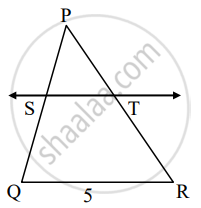Q.5 | Q 2

An architecture have model of building. Length of building is 1 m then length of model is 0.75 cm. Then find length and height of model building whose actual length is 22.5 m and height is 10 m

Advertisement Remove all ads

## Chapter 1: Similarity

Q.1 (A)Q.1 (B)Q.2 (A)Q.2 (B)Q.3 (A)Q.3 (B)Q.4Q.5## SCERT Maharashtra Question Bank solutions for 10th Standard SSC Mathematics 2 Geometry Maharashtra State Board 2021 chapter 1 - Similarity

SCERT Maharashtra Question Bank solutions for 10th Standard SSC Mathematics 2 Geometry Maharashtra State Board 2021 chapter 1 (Similarity) include all questions with solution and detail explanation. This will clear students doubts about any question and improve application skills while preparing for board exams. The detailed, step-by-step solutions will help you understand the concepts better and clear your confusions, if any. Shaalaa.com has the Maharashtra State Board 10th Standard SSC Mathematics 2 Geometry Maharashtra State Board 2021 solutions in a manner that help students grasp basic concepts better and faster.

Further, we at Shaalaa.com provide such solutions so that students can prepare for written exams. SCERT Maharashtra Question Bank textbook solutions can be a core help for self-study and acts as a perfect self-help guidance for students.

Concepts covered in 10th Standard SSC Mathematics 2 Geometry Maharashtra State Board 2021 chapter 1 Similarity are Properties of Ratios of Areas of Two Triangles, Similarity of Triangles, Similar Triangles, Similarity Triangle Theorem, Areas of Two Similar Triangles, Property of three parallel lines and their transversals, Property of an Angle Bisector of a Triangle, Basic Proportionality Theorem Or Thales Theorem, Converse of Basic Proportionality Theorem, Appolonius Theorem, Application of Pythagoras Theorem in Acute Angle and Obtuse Angle, Areas of Similar Triangles, Similarity.

Using SCERT Maharashtra Question Bank 10th Standard [इयत्ता १० वी] solutions Similarity exercise by students are an easy way to prepare for the exams, as they involve solutions arranged chapter-wise also page wise. The questions involved in SCERT Maharashtra Question Bank Solutions are important questions that can be asked in the final exam. Maximum students of Maharashtra State Board 10th Standard [इयत्ता १० वी] prefer SCERT Maharashtra Question Bank Textbook Solutions to score more in exam.

Get the free view of chapter 1 Similarity 10th Standard [इयत्ता १० वी] extra questions for 10th Standard SSC Mathematics 2 Geometry Maharashtra State Board 2021 and can use Shaalaa.com to keep it handy for your exam preparation

Advertisement Remove all ads
Share
Notifications

View all notifications

Forgot password?